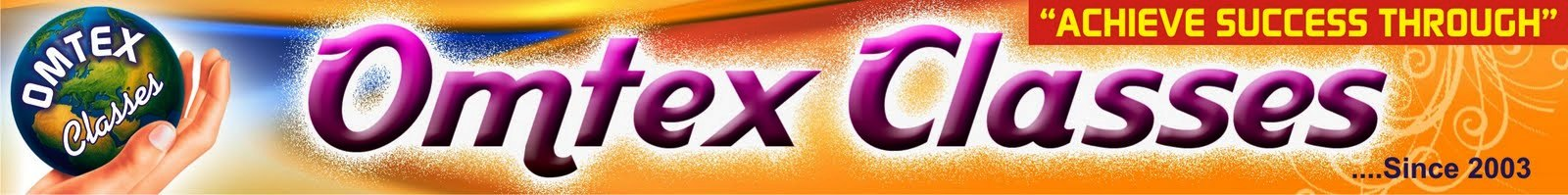9TH MAHARASHTRA: MATHS I 10TH TAMIL NADU: MATHS, SCIENCE, SSLC 10TH NEW TEXT BOOK PDF DOWNLOAD CBSE 10TH: MATHS 2019 BOARD PAPER SOLUTION: 2019 BOARD PAPER SOLUTION

Science I Revision, Fill in the blanks.Science I Revision,
Fill in the blanks.
Gravitation
1. The orbit of a planet is an ellipse with the Sun at one of the foci.
2. According to Newton's first law, mass is the measure of inertia of an object.
3. True free fall is possible only in vacuum.
4. An object going vertically upwards will be free from the gravitational influence of the earth, it its initial velocity is equal to escape velocity of the earth.
Periodic Classification of Elements.
5. Elements lithium, sodium and potassium make Dobereiner's triad.
6. According to periodic law of Mendeleev, the properties of elements are periodic function of their atomic masses.
7. The lightest element in the modern periodic table is hydrogen.
8. The first element of group 15 in the modern periodic table is nitrogen.
9. Atomic number (Z) of an element is the number of protons present in the nucleus of an atom of that element.
10. Iodine (I2) exists as solid at room temperature.
Chemical Reactions and Equations.
11. The composition of matter changes in a chemical change.
12. Evaporation of water is a physical change.
13. When electric current is passed through acidulated water, electrolysis of water takes place.
14. Addition of an aqueous solution of ZnSO4 to an aqueous solution of BaCl2 is an example of double displacement reaction.
15. The conversion of ferrous sulphate to ferric sulphate is oxidation reaction.
16. Damage of a metal due to oxidation by atmospheric components is called corrosion.
17. The rust chemically is Fe2O3.xH2O.
18. To prevent rusting, a layer of zinc metal is applied on iron sheets.
Effects of Electric Current.
19. The live and neutral wires have potential difference of 220 V.
20. A copper wire with a resistive coating when is wound in a chain of loops is called solenoid.